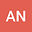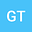loading page

COVID-19 transmission risk factors
••• Alessio Notari,
• Giorgio Torrieri
Alessio Notari
University of Barcelona
Author ProfileGiorgio Torrieri
State University of Campinas
Author Profile## Abstract

We analyze risk factors correlated with the initial transmission growth rate of the recent COVID-19 pandemic in different countries. The number of cases follows in its early stages an almost exponential expansion; we chose as a starting point in each country the first day $d_i$ with 30 cases and we fitted for 12 days, capturing thus the early exponential growth. We looked then for linear correlations of the exponents $\alpha$ with other variables, for a sample of 126 countries. We find a positive correlation, {\it i.e. faster spread of COVID-19}, with high confidence level with the following variables, with respective $p$-value: low Temperature ($4\cdot10^{-7}$), high ratio of old vs.~working-age people ($3\cdot10^{-6}$), life expectancy ($8\cdot10^{-6}$), number of international tourists ($1\cdot10^{-5}$), earlier epidemic starting date $d_i$ ($2\cdot10^{-5}$), high level of physical contact in greeting habits ($6 \cdot 10^{-5}$), lung cancer prevalence ($6 \cdot 10^{-5}$), obesity in males ($1 \cdot 10^{-4}$), share of population in urban areas ($2\cdot10^{-4}$), cancer prevalence ($3 \cdot 10^{-4}$), alcohol consumption ($0.0019$), daily smoking prevalence ($0.0036$), UV index ($0.004$, smaller sample, 73 countries), low Vitamin D serum levels ($0.002-0.006$, smaller sample, $\sim 50$ countries). There is highly significant correlation also with blood type: positive correlation with types RH- ($3\cdot10^{-5}$) and A+ ($3\cdot10^{-3}$), negative correlation with B+ ($2\cdot10^{-4}$). We also find positive correlation with moderate confidence level ($p$-value of $0.02\sim0.03$) with: CO$_2$/SO emissions, type-1 diabetes in children, low vaccination coverage for Tuberculosis (BCG). Several of the above variables are correlated with each other and likely to have common interpretations. We thus performed a Principal Component Analysis, in order to find the significant independent linear combinations of such variables. We also analyzed the possible existence of a bias: countries with low GDP-per capita, typically located in warm regions, might have less intense testing and we discuss correlation with the above variables